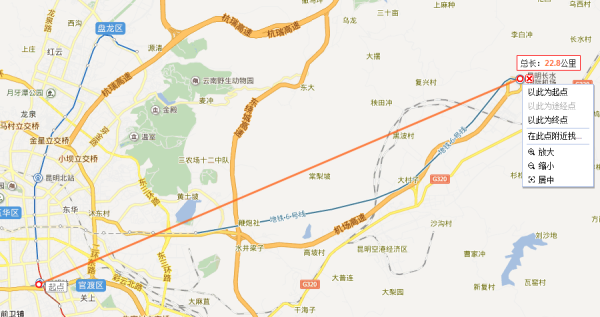## 计算地图经纬度之间两点之间的距离

•
• 4224
• PHP
• 1
• super_dodo
• 2014/06/11

```//求两者经纬度之间的距离
function getDistance(\$lat1,\$lng1,\$lat2,\$lng2){
//将角度转为狐度
return round(\$s);
}

//示例
//102.727775,25.093923  ---龙泉路财经大学
//102.728493,25.019685  ----昆明火车站
//102.936038,25.10086   ----长水机场
//102.774774,24.962569  ----星耀路
\$lat1 = 25.019685;   //昆明火车站纬度
\$lng1 = 102.728493;  //昆明火车站经度
\$lat2 = 25.10086;    //长水机场纬度
\$lng2 = 102.936038;  //长水机场经度

\$long = \$this->getDistance(\$lat1,\$lng1,\$lat2,\$lng2);
//昆明火车站到长水机场得到计算的结果为 22.796
//百度地图上量出来的为22.8km
//基本吻合 ---见下图
``````BEGIN
#Routine body goes here...
set @num=ROUND((2 * 6378.137* ASIN(SQRT(POW(SIN(PI()*(lat1-lat2)/360), 2)+COS(PI()*lat1/180)* COS(lat2*PI()/180) * POW(SIN(PI()*(lng1-lng2)/360), 2)))),1);
RETURN @num;
END

//计算的时候sql
\$sql="SELECT id,lat,lng,get_distance(\$ulng,\$ulat,lng,lat) as distance FROM company;
```